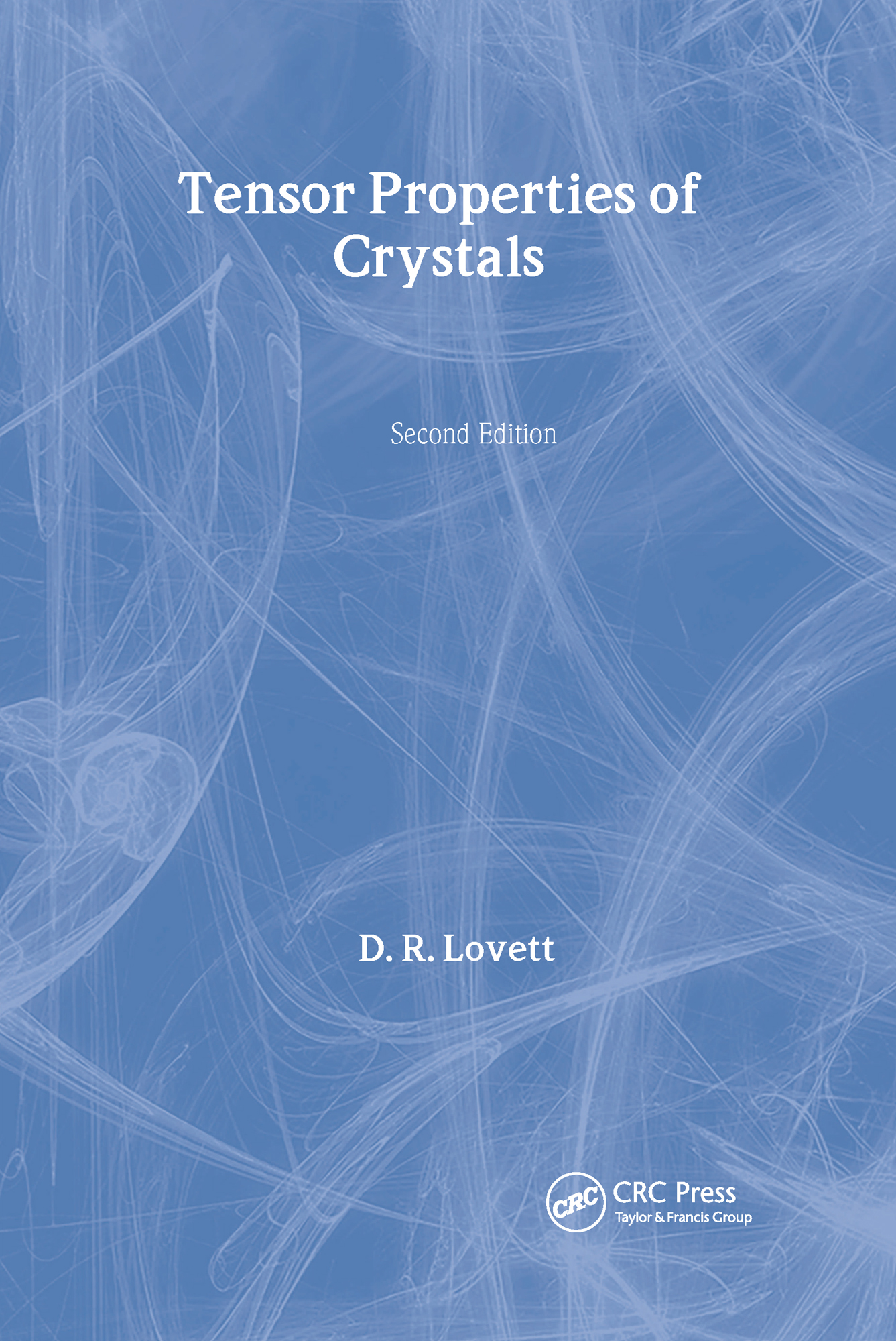Tensor Properties of Crystals

2nd Edition

CRC Press

176 pages

Purchasing Options:\$ = USD
Paperback: 9780750306263
pub: 1999-01-01
SAVE ~\$13.79
\$68.95
\$55.16
x
Hardback: 9780750306256
pub: 1999-01-01
SAVE ~\$46.00
\$230.00
\$184.00
x
eBook (VitalSource) : 9780203737286
pub: 2018-05-04
from \$31.48

FREE Standard Shipping!

Description

The use of single crystals for scientific and technological applications is now widespread in solid-state physics, optics, electronics, materials science, and geophysics. An understanding of the variation of physical properties with crystalline direction is essential to maximize the performance of solid-state devices.

Written from a physical viewpoint and avoiding advanced mathematics, Tensor Properties of Crystals provides a concise introduction to the tensor properties of crystals at a level suitable for advanced undergraduate and graduate students. While retaining the successful basic format of the well-known first edition, this second edition brings the material up to date with the latest developments in nonlinear optics and modulated structures. Because of the increasing importance of nonlinear optics, a new chapter on optoelectronics has been added. This edition also includes a short discussion on incommensurate modulated structures in the final chapter because they are relevant to high temperature superconductors and to ferroelectric and ferromagnetic materials. The book extensively contains diagrams, worked examples, and problems with answers throughout.

Reviews

Praise for the first edition

"An excellent introduction to the use of tensors in solid state physics…extremely good value for money."

-Australian & New Zealand Physicist

"Simple and clear presentation…suitable for teaching crystal physics as a subsidiary subject."

-Crystal Research and Technology

"The book provides a concise introduction to the tensor properties of crystals at a level suitable for advanced undergraduate students."

-Zentralblatt, vol. 988

CRYSTALS AND CRYSTAL SYMMETRY

Structure of solids. Close-packing structures. Two-dimensional lattices. Three-dimensional lattices. Crystallographic indices for planes-Miller indices. Crystallographic direction indices. Worked examples on symmetry problems. Problems.

INTRODUCING TENSORS

Introduction to the notation. Reducing the number of components. Transformation of axes. Transformation of a vector. Transformation of the coordinates of a point. Transformation and definition of a tensor. Second-rank symmetrical tensors-the representation quadric. Neumann's principle. Some examples of tensors. Worked examples showing change of axes for a tensor. Problem.

SECOND-RANK TENSORS-CONDUCTIVITY

Thermal conductivity and thermal resistivity. Heat flow in crystal samples. The radius-normal property of the representation quadric. Electrical conductivity and electrical resistivity. Diffusion. Some worked examples of second-rank tensor properties. Problems.

FOURTH-RANK TENSORS-ELASTICITY

Introduction. Strain. Symmetrical and antisymmetrical tensors. The strain tensor. Stress. Elasticity. The matrix notation. Effect of crystal symmetry-equating components by inspection. Elasticity components in cubic crystals and polycrystalline samples. Elasticity components in other crystal systems. Worked examples on stress, strain and elasticity. Problems.

CRYSTAL OPTICS

Introduction. The indicatrix. The wave surface. Biaxial crystals. Double refraction (birefringence) at a boundary. Worked examples on polarization and birefringence. Problems.

AXIAL TENSORS

Definition of an axial tensor. Transformation of axial vectors. Transformation of axial tensors. Optical activity. Optical activity in the presence of birefringence. The gyration tensor-second-rank axial tensor. The Hall effect-third-rank axial tensor. The Hall effect- relationship to symmetry. Magnetoresistance and other effects. Problems.

FURTHER TENSOR APPLICATIONS

Introduction. Thermal expansion. The pyroelectric effect. Piezoelectricity. Photoelasticity. The linear electro-optic effect (the Pockels effect). The quadratic electro-optic effect (the Kerr effect). Some concluding comments. Problems.

APPENDICES

REFERENCES AND FURTHER READING

INDEX